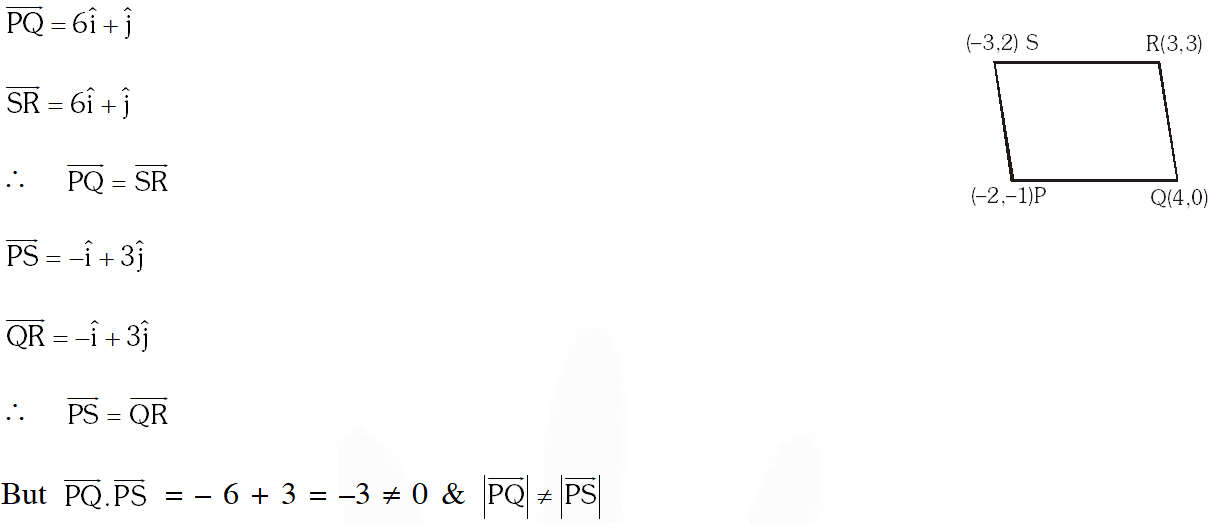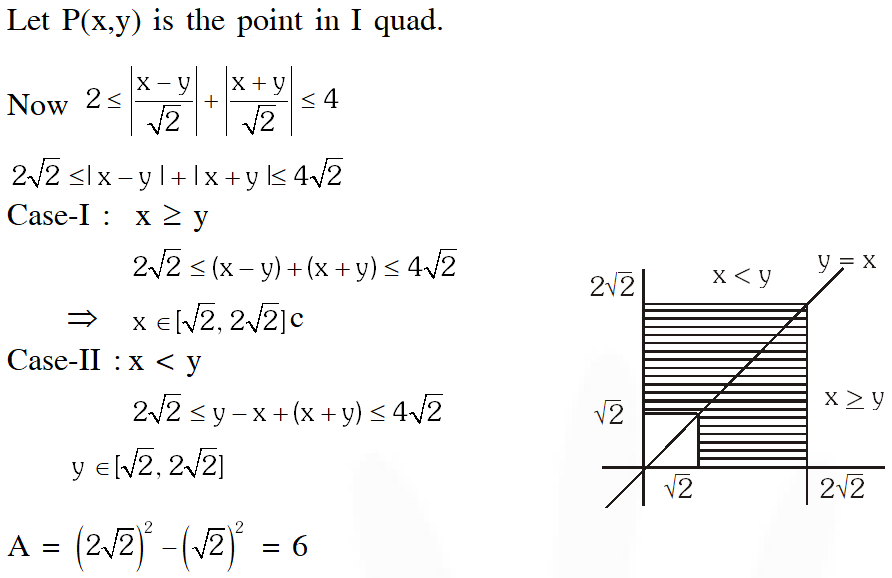Most Affordable JEE | NEET | 8,9,10 Preparation by Kota's Top IITian Doctor Faculties

# Straight Line - JEE Advanced Previous Year Questions with SolutionsJEE Advanced Previous Year Questions of Math with Solutions are available at eSaral. Practicing JEE Advanced Previous Year Papers Questions of mathematics will help the JEE aspirants in realizing the question pattern as well as help in analyzing weak & strong areas. eSaral helps the students in clearing and understanding each topic in a better way. eSaral also provides complete chapter-wise notes of Class 11th and 12th both for all subjects. Besides this, eSaral also offers NCERT Solutions, Previous year questions for JEE Main and Advance, Practice questions, Test Series for JEE Main, JEE Advanced and NEET, Important questions of Physics, Chemistry, Math, and Biology and many more. Download eSaral app for free study material and video tutorials.
Q. Let $\mathrm{P}, \mathrm{Q}, \mathrm{R}$ and $\mathrm{S}$ be the points on the plane with position vectors $-2 \hat{\mathrm{i}}-\hat{\mathrm{j}}, 4 \hat{\mathrm{i}}, 3 \hat{\mathrm{i}}+3 \hat{\mathrm{j}}$ and $-3 \hat{\mathrm{j}}$ and $-3 \hat{\mathrm{i}}+2 \hat{\mathrm{j}}$ respectively. The quadrilateral PQRS must be a (A) parallelogram, which is neither a rhombus nor a rectangle (B) square (C) rectangle, but not a square (D) rhombus, but not a square [JEE 2010, 3]
Ans. (A)$\Rightarrow$ PQRS is a parallelogram but neither a rhombus nor a rectangle.
Q. A straight line L through the point $(3,-2)$ is inclined at an angle $60^{\circ}$ to the line $\sqrt{3} x+y=1$. If $L$ also intersect the x-axis, then the equation of $L$ is (A) $y+\sqrt{3} x+2-3 \sqrt{3}=0$ (B) $\mathrm{y}-\sqrt{3} \mathrm{x}+2+3 \sqrt{3}=0$ (C) $\sqrt{3} y-x+3+2 \sqrt{3}=0$ (D) $\sqrt{3} y+x-3+2 \sqrt{3}=0$ [JEE 2011, 3 (–1)]
Ans. (B)Q. For a > b > c > 0, the distance between (1, 1) and the point of intersection of the lines $a x+b y+c=0$ and $b x+a y+c=0$ is less than $2 \sqrt{2} .$ Then (A) a + b – c > 0 (B) a – b + c < 0 (C) a – b + c > 0 (D) a + b – c < 0 [JEE-Advanced 2013, 2]
Ans. (A or C or A,C) Point of intersection of both lines is $\left(-\frac{c}{(a+b)},-\frac{c}{(a+b)}\right)$ Distance between $\left(-\frac{c}{(a+b)},-\frac{c}{(a+b)}\right) \&(1,1)$ is Distance $=\sqrt{\frac{(a+b+c)^{2}}{(a+b)^{2}} \times 2}<2 \sqrt{2}$ $a+b+c<2(a+b)$ $a+b-c>0$ According to given condition option (C) also correct.
Q. For a point $P$ in the plane, let $d_{1}(P)$ and $d_{2}(P)$ be the distances of the point $P$ from the lines $x-y=0$ and $x+y=0$ respectively. The area of the region $R$ consisting of all points $P$ lying in the first quadrant of the plane and satisfying $2 \leq d_{1}(P)+d_{2}(P) \leq 4,$ is [JEE(Advanced)-2014, 3]
Ans. 6Kya
Oct. 29, 2023, 6:35 a.m.
Kya
arun kumar
July 30, 2022, 7:55 a.m.
making fool
sanchit
July 22, 2022, 10:30 p.m.
kyu
Sept. 15, 2021, 3:28 p.m.
kyu
Shruti Agarwal
Sept. 4, 2021, 8:52 a.m.
Please edit all questions of jee advanced ..that will be more useful.....
Keshav
May 11, 2021, 8:14 p.m.
I suggest esaral to add more questions. I am unsatisfied from this😑.
Lakshay Khandelwal
March 31, 2021, 8:25 p.m.
Very few but challenging questions please edit more questions 👍
Samael
Oct. 13, 2020, 1:07 a.m.
Btwlast question is fantastic..
Samael
Oct. 13, 2020, 12:44 a.m.
Is that it? ...only this much questions from straight lines ?